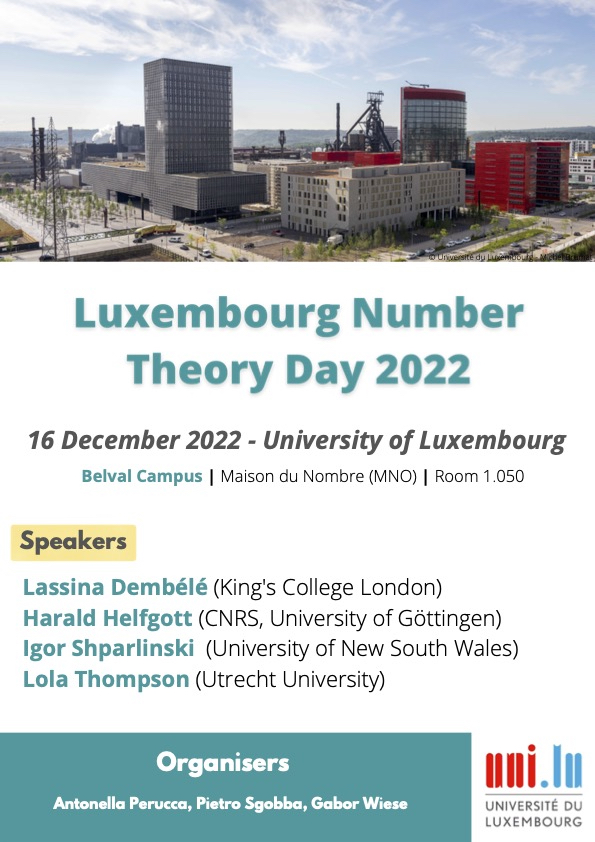# Luxembourg Number Theory Day 2022The Luxembourg Number Theory Day will take place on December 16, 2022, on the Esch-Belval Campus of the University of Luxembourg.

A Webex link to attend the talks remotely will be sent upon request. Please let us also know if you plan to attend in person.

### Speakers

Lassina Dembélé (King's College London)

Harald Helfgott (CNRS, University of Göttingen)

Igor Shparlinski (University of New South Wales)

Lola Thompson (Utrecht University)

### Contacts

Antonella Perucca, Pietro Sgobba, Gabor Wiese. Email addresses: name.surname at uni.lu

### Location

All of the talks will take place in room MNO 1.050 on the 1st floor of the Maison du Nombre at 6, Avenue de la Fonte, Esch-sur Alzette. You can see this on Google Maps or OpenStreetMap.

### Schedule

 Time Speaker Title 9:30-10:10 Igor Shparlinski Integers of prescribed arithmethic structure in residue classes 10:10-10:30 Coffee break 10:30-11:10 Lassina Dembélé Explicit inertial Langlands correspondence for ${\rm GL}_2$ and arithmetic applications 11:20-12:00 Lola Thompson Summing μ(n): an even faster elementary algorithm 12:00-13:00 Lunch break 13:00-13:40 Harald Helfgott Expansion, divisibility and parity

### Abstracts

Igor Shparlinski (University of New South Wales) Integers of prescribed arithmethic structure in residue classes

We give an overview of recent results about the distribution of some special integers in residues classes modulo a large integer $q$. Questions of this type were introduced by Erdos, Odlyzko and Sarkozy (1987), who considered products of two primes as a relaxation of the classical question about the distribution of primes in residue classes. Since that time, numerous variations have appeared for different sequences of integers. The types of numbers we discuss include smooth, square-free, square-full and almost primes integers.

We also expose, without going into technical details, the wealth of different techniques behind these results: sieve methods, bounds of short Kloosterman sums, bounds of short character sums and many others.

Lassina Dembélé (King's College London) Explicit inertial Langlands correspondence for ${\rm GL}_2$ and arithmetic applications

In this talk we will describe an algorithm for computing automorphic types for ${\rm GL}_2$. Then, we will give several arithmetic applications. (This is joint work with Nuno Freitas and John Voight.)

Lola Thompson (Utrecht University) Summing μ(n): an even faster elementary algorithm

We present a new algorithm for computing $M(x) = \sum_{n \leq x} \mu(n)$, where $\mu(n)$ is the Möbius function. Our algorithm takes
$time O_\epsilon( x^{3/5} (\log x)^{8/5+\epsilon} ) and space O( x^{3/10} (\log x)^{13/10} ),$
where time and space are measured bitwise. This is the first improvement in the exponent of $x$ for an elementary algorithm since 1985. While there is an analytic algorithm due to Lagarias-Odlyzko with computations based on the integrals of $\zeta(s)$ that only takes time $O(x^{1/2 + \epsilon})$, our algorithm has the advantage of being easier to implement. The new approach roughly amounts to analyzing the difference between a model that we obtain via Diophantine approximation and reality, and showing that it has a simple description in terms of congruence classes and segments. This talk is based on joint work with Harald Andrés Helfgott.

Harald Helfgott (CNRS, University of Göttingen) Expansion, divisibility and parity

We will discuss a graph that encodes the divisibility properties of integers by primes. We prove that this graph has a strong local expander property almost everywhere. We then obtain several consequences in number theory, beyond the traditional parity barrier, by combining our result with Matomaki-Radziwill. For instance: for lambda the Liouville function (that is, the completely multiplicative function with lambda(p) = -1 for every prime), (1/\log x) \sum_{n\leq x} lambda(n) \lambda(n+1)/n = O(1/sqrt(log log x)), which is stronger than well-known results by Tao and Tao-Teravainen. We also manage to prove, for example, that lambda(n+1) averages to 0 at almost all scales when n restricted to have a specific number of prime divisors Omega(n)=k, for any "popular" value of k (that is, k = log log N + O(sqrt(log log N)) for n<=N).